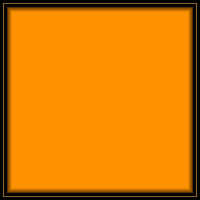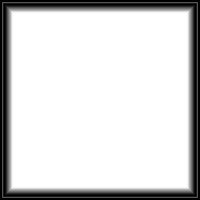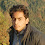## Friday, February 3, 2012

### Draw a 3D Rubik cube using OpenGL utility toolkits GLUT with source code

This article is similar  to my previous article drawing 3D chess board.To make Rubik cube real I have used texture mapping.At first I draw a one cube where I mapped texture in all six side of cube with six different color images and you can save these images from here.After that 27 cube is drawn by looping and final Rubik cube is drawn, rotating in (2,2,0) axis.For texture mapping we have to write a code to load a images and store a value of (R1,G1,B1,R2,G2,B2,……………)pixels in pixels array.In this project there is a Image class where you can see in source code below in file name imageloader.h and imageloader.cpp which I have take from a website www.videotutorialsrock.com.
All the images for this program is given below save it in your project file location.To compile this code don’t forget to setup GLUT in codeblocks or visual studio.

Here is the source code separately with separate file name, copy and save code with respective file name.
main.cpp
`#include<windows.h>`
`#include <glut.h>`
`#include <cstdlib>`
`#include <iostream>`
`#include <fstream>`
`#include <cmath>`
` `
`#include "imageloader.h"`
`using namespace std;`
`float _angle = 0;`
`float i;`
`int k=0;`
`const float BOX_SIZE = 2.0f;`
`GLuint white_textureId;`
`GLuint red_textureId;`
`GLuint blue_textureId;`
`GLuint green_textureId;`
`GLuint yellow_textureId;`
`GLuint orange_textureId;`
`GLuint _displayListId_smallcube; //The OpenGL id of the display list`
`//Makes the image into a texture, and returns the id of the texture`
`GLuint loadTexture(Image* image) {`
`    GLuint textureId;`
`    glGenTextures(1, &textureId);`
`    glBindTexture(GL_TEXTURE_2D, textureId);`
`    glTexImage2D(GL_TEXTURE_2D,`
`                 0,`
`                 GL_RGB,`
`                 image->width, image->height,`
`                 0,`
`                 GL_RGB,`
`                 GL_UNSIGNED_BYTE,`
`                 image->pixels);`
`    return textureId;`
`}`
`void handleKeypress(unsigned char key,int x,int y)`
`{`
`    switch(key)`
`    {`
`        case 27:`
`        exit(0);`
`    }`
`}`
` `
`void handleResize(int w,int h)`
`{`
`    glViewport(0,0,w,h);`
`    glMatrixMode(GL_PROJECTION);`
`    glLoadIdentity();`
`    gluPerspective(45.0,(double)w/(double)h,1.0,200);`
`    gluLookAt(0.0f,5.5f, 15.0f,`
`              0.0f,0.0f,0.0f,`
`              0.0f,1.0f,0.0f);`
`}`
` `
`void draw_smallcube()`
`{`
` `
`    Image* image = loadBMP("white.bmp");`
`    white_textureId = loadTexture(image);`
`    delete image;`
` `
`        Image* image1 = loadBMP("red.bmp");`
`    red_textureId = loadTexture(image1);`
`    delete image1;`
` `
`        Image* image2 = loadBMP("blue.bmp");`
`    blue_textureId = loadTexture(image2);`
`    delete image2;`
` `
`        Image* image3 = loadBMP("orange.bmp");`
`    orange_textureId = loadTexture(image3);`
`    delete image3;`
` `
`        Image* image4 = loadBMP("green.bmp");`
`    green_textureId = loadTexture(image4);`
`    delete image4;`
` `
`        Image* image5 = loadBMP("yellow.bmp");`
`    yellow_textureId = loadTexture(image5);`
`    delete image5;`
` `
`  `
`     glEnable(GL_TEXTURE_2D);`
`     glBindTexture(GL_TEXTURE_2D, orange_textureId);`
`     glTexParameteri(GL_TEXTURE_2D, GL_TEXTURE_MIN_FILTER, GL_LINEAR);`
`     glTexParameteri(GL_TEXTURE_2D, GL_TEXTURE_MAG_FILTER, GL_LINEAR);`
` `
`    glBegin(GL_QUADS);`
` `
`    //Top face`
`    //glNormal3f(0.0, 1.0f, 0.0f);`
`    glTexCoord2f(0.0f, 0.0f);`
`    glVertex3f(-BOX_SIZE / 2, BOX_SIZE / 2, -BOX_SIZE / 2);`
`    glTexCoord2f(1.0f, 0.0f);`
`    glVertex3f(-BOX_SIZE / 2, BOX_SIZE / 2, BOX_SIZE / 2);`
`    glTexCoord2f(1.0f, 1.0f);`
`    glVertex3f(BOX_SIZE / 2, BOX_SIZE / 2, BOX_SIZE / 2);`
`    glTexCoord2f(0.0f, 1.0f);`
`    glVertex3f(BOX_SIZE / 2, BOX_SIZE / 2, -BOX_SIZE / 2);`
` `
`    glEnd();`
`    glDisable(GL_TEXTURE_2D);`
` `
` `
`    glEnable(GL_TEXTURE_2D);`
`    glBindTexture(GL_TEXTURE_2D, red_textureId);`
`    glTexParameteri(GL_TEXTURE_2D, GL_TEXTURE_MIN_FILTER, GL_LINEAR);`
`    glTexParameteri(GL_TEXTURE_2D, GL_TEXTURE_MAG_FILTER, GL_LINEAR);`
` `
`    glBegin(GL_QUADS);`
`    //Bottom face`
` `
`    //glNormal3f(0.0, -1.0f, 0.0f);`
`    glTexCoord2f(0.0f, 0.0f);`
`    glVertex3f(-BOX_SIZE / 2, -BOX_SIZE / 2, -BOX_SIZE / 2);`
`    glTexCoord2f(1.0f, 0.0f);`
`    glVertex3f(BOX_SIZE / 2, -BOX_SIZE / 2, -BOX_SIZE / 2);`
`    glTexCoord2f(1.0f, 1.0f);`
`    glVertex3f(BOX_SIZE / 2, -BOX_SIZE / 2, BOX_SIZE / 2);`
`    glTexCoord2f(0.0f, 1.0f);`
`    glVertex3f(-BOX_SIZE / 2, -BOX_SIZE / 2, BOX_SIZE / 2);`
` `
`    glEnd();`
` `
` `
`    glEnable(GL_TEXTURE_2D);`
`    glBindTexture(GL_TEXTURE_2D, green_textureId);`
`    glTexParameteri(GL_TEXTURE_2D, GL_TEXTURE_MIN_FILTER, GL_LINEAR);`
`    glTexParameteri(GL_TEXTURE_2D, GL_TEXTURE_MAG_FILTER, GL_LINEAR);`
` `
`    glBegin(GL_QUADS);`
` `
`    //Left face`
`    //glNormal3f(-1.0, 0.0f, 0.0f);`
`    glTexCoord2f(0.0f, 0.0f);`
`    glVertex3f(-BOX_SIZE / 2, -BOX_SIZE / 2, -BOX_SIZE / 2);`
`    glTexCoord2f(1.0f, 0.0f);`
`    glVertex3f(-BOX_SIZE / 2, -BOX_SIZE / 2, BOX_SIZE / 2);`
`    glTexCoord2f(1.0f, 1.0f);`
`    glVertex3f(-BOX_SIZE / 2, BOX_SIZE / 2, BOX_SIZE / 2);`
`    glTexCoord2f(0.0f, 1.0f);`
`    glVertex3f(-BOX_SIZE / 2, BOX_SIZE / 2, -BOX_SIZE / 2);`
` `
`    glEnd();`
` `
` `
`    glEnable(GL_TEXTURE_2D);`
`    glBindTexture(GL_TEXTURE_2D, blue_textureId);`
`    glTexParameteri(GL_TEXTURE_2D, GL_TEXTURE_MIN_FILTER, GL_LINEAR);`
`    glTexParameteri(GL_TEXTURE_2D, GL_TEXTURE_MAG_FILTER, GL_LINEAR);`
` `
`    glBegin(GL_QUADS);`
` `
`    //Right face`
`    //glNormal3f(1.0, 0.0f, 0.0f);`
`    glTexCoord2f(0.0f, 0.0f);`
`    glVertex3f(BOX_SIZE / 2, -BOX_SIZE / 2, -BOX_SIZE / 2);`
`    glTexCoord2f(1.0f, 0.0f);`
`    glVertex3f(BOX_SIZE / 2, BOX_SIZE / 2, -BOX_SIZE / 2);`
`    glTexCoord2f(1.0f, 1.0f);`
`    glVertex3f(BOX_SIZE / 2, BOX_SIZE / 2, BOX_SIZE / 2);`
`    glTexCoord2f(0.0f, 1.0f);`
`    glVertex3f(BOX_SIZE / 2, -BOX_SIZE / 2, BOX_SIZE / 2);`
` `
`    glEnd();`
` `
`    glEnable(GL_TEXTURE_2D);`
`    glBindTexture(GL_TEXTURE_2D, white_textureId);`
`    glTexParameteri(GL_TEXTURE_2D, GL_TEXTURE_MIN_FILTER, GL_LINEAR);`
`    glTexParameteri(GL_TEXTURE_2D, GL_TEXTURE_MAG_FILTER, GL_LINEAR);`
` `
`    glBegin(GL_QUADS);`
` `
`    //Front face`
`    //glNormal3f(0.0, 0.0f, 1.0f);`
`    glTexCoord2f(0.0f, 0.0f);`
`    glVertex3f(-BOX_SIZE / 2, -BOX_SIZE / 2, BOX_SIZE / 2);`
`    glTexCoord2f(1.0f, 0.0f);`
`    glVertex3f(BOX_SIZE / 2, -BOX_SIZE / 2, BOX_SIZE / 2);`
`    glTexCoord2f(1.0f, 1.0f);`
`    glVertex3f(BOX_SIZE / 2, BOX_SIZE / 2, BOX_SIZE / 2);`
`    glTexCoord2f(0.0f, 1.0f);`
`    glVertex3f(-BOX_SIZE / 2, BOX_SIZE / 2, BOX_SIZE / 2);`
` `
`    glEnd();`
` `
` `
`    glEnable(GL_TEXTURE_2D);`
`    glBindTexture(GL_TEXTURE_2D, yellow_textureId);`
`    glTexParameteri(GL_TEXTURE_2D, GL_TEXTURE_MIN_FILTER, GL_LINEAR);`
`    glTexParameteri(GL_TEXTURE_2D, GL_TEXTURE_MAG_FILTER, GL_LINEAR);`
` `
`    glBegin(GL_QUADS);`
`    //Back face`
`    //glNormal3f(0.0, 0.0f, -1.0f);`
`    glTexCoord2f(0.0f, 0.0f);`
`    glVertex3f(-BOX_SIZE / 2, -BOX_SIZE / 2, -BOX_SIZE / 2);`
`    glTexCoord2f(1.0f, 0.0f);`
`    glVertex3f(-BOX_SIZE / 2, BOX_SIZE / 2, -BOX_SIZE / 2);`
`    glTexCoord2f(1.0f, 1.0f);`
`    glVertex3f(BOX_SIZE / 2, BOX_SIZE / 2, -BOX_SIZE / 2);`
`    glTexCoord2f(0.0f, 1.0f);`
`    glVertex3f(BOX_SIZE / 2, -BOX_SIZE / 2, -BOX_SIZE / 2);`
` `
`    glEnd();`
`    //glDisable(GL_TEXTURE_2D);`
` `
`     //glutSwapBuffers();`
`}`
` `
` `
`void initRendering()`
`{`
`    glEnable(GL_DEPTH_TEST);`
`    glEnable(GL_COLOR_MATERIAL);`
`    //glEnable(GL_NORMALIZE);`
`    //glEnable(GL_COLOR_MATERIAL);`
`    glClearColor(0.0f,0.0f,0.2f,1.0f);`
`    glHint(GL_PERSPECTIVE_CORRECTION_HINT, GL_NICEST);`
` `
`    `
` `
` `
`    //Set up a display list for drawing a cube`
`    _displayListId_smallcube = glGenLists(1); //Make room for the display list`
`    glNewList(_displayListId_smallcube, GL_COMPILE); //Begin the display list`
`    draw_smallcube(); //Add commands for drawing a black area to the display list`
`    glEndList(); //End the display list`
` `
` `
`}`
`void drawScene()`
`{`
`    glClear(GL_COLOR_BUFFER_BIT|GL_DEPTH_BUFFER_BIT);`
`    glMatrixMode(GL_MODELVIEW);`
`    glLoadIdentity();`
`    glTranslatef(0,-5,-10);`
`    glRotatef(-_angle, 2.0f, 2.0f, 0.0f);`
`    `
`    for(int i=0;i<5;i+=2)`
`    {`
`        for(int j=0;j<5;j+=2)`
`        {`
`            for(int k=0;k<5;k+=2)`
`            {`
`            glPushMatrix();`
`            glTranslatef(i,j,k);`
`            glCallList(_displayListId_smallcube);`
`            glPopMatrix();`
`            }`
`        }`
`    }`
` `
`    glutSwapBuffers();`
` `
` `
` `
`}`
`void update(int value) {`
`    _angle += 2.0f;`
`    if (_angle > 360) {`
`        _angle -= 360;`
`    }`
`    glutPostRedisplay();`
`    glutTimerFunc(10, update, 0);`
`}`
`int main(int argc,char** argv)`
`{`
`    glutInit(&argc,argv);`
`    glutInitDisplayMode(GLUT_DOUBLE|GLUT_RGB|GLUT_DEPTH);`
`    glutInitWindowSize(800,800);`
` `
`    glutCreateWindow("basic shape");`
`    initRendering();`
`    glutFullScreen();`
` `
`    glutDisplayFunc(drawScene);`
`    glutKeyboardFunc(handleKeypress);`
`    glutReshapeFunc(handleResize);`
`    glutTimerFunc(25, update, 0);`
` `
`    glutMainLoop();`
` `
`    return 0;`
` `
`}`
` `

` `
`#include <assert.h>`
`#include <fstream>`
` `
`#include "imageloader.h"`
` `
`using namespace std;`
` `
`Image::Image(char* ps, int w, int h) : pixels(ps), width(w), height(h) {`
`    `
`}`
` `
`Image::~Image() {`
`    delete[] pixels;`
`}`
` `
`namespace {`
`    //Converts a four-character array to an integer, using little-endian form`
`    int toInt(const char* bytes) {`
`        return (int)(((unsigned char)bytes << 24) |`
`                     ((unsigned char)bytes << 16) |`
`                     ((unsigned char)bytes << 8) |`
`                     (unsigned char)bytes);`
`    }`
`    `
`    //Converts a two-character array to a short, using little-endian form`
`    short toShort(const char* bytes) {`
`        return (short)(((unsigned char)bytes << 8) |`
`                       (unsigned char)bytes);`
`    }`
`    `
`    //Reads the next four bytes as an integer, using little-endian form`
`    int readInt(ifstream &input) {`
`        char buffer;`
`        input.read(buffer, 4);`
`        return toInt(buffer);`
`    }`
`    `
`    //Reads the next two bytes as a short, using little-endian form`
`    short readShort(ifstream &input) {`
`        char buffer;`
`        input.read(buffer, 2);`
`        return toShort(buffer);`
`    }`
`    `
`    //Just like auto_ptr, but for arrays`
`    template<class T>`
`    class auto_array {`
`        private:`
`            T* array;`
`            mutable bool isReleased;`
`        public:`
`            explicit auto_array(T* array_ = NULL) :`
`                array(array_), isReleased(false) {`
`            }`
`            `
`            auto_array(const auto_array<T> &aarray) {`
`                array = aarray.array;`
`                isReleased = aarray.isReleased;`
`                aarray.isReleased = true;`
`            }`
`            `
`            ~auto_array() {`
`                if (!isReleased && array != NULL) {`
`                    delete[] array;`
`                }`
`            }`
`            `
`            T* get() const {`
`                return array;`
`            }`
`            `
`            T &operator*() const {`
`                return *array;`
`            }`
`            `
`            void operator=(const auto_array<T> &aarray) {`
`                if (!isReleased && array != NULL) {`
`                    delete[] array;`
`                }`
`                array = aarray.array;`
`                isReleased = aarray.isReleased;`
`                aarray.isReleased = true;`
`            }`
`            `
`            T* operator->() const {`
`                return array;`
`            }`
`            `
`            T* release() {`
`                isReleased = true;`
`                return array;`
`            }`
`            `
`            void reset(T* array_ = NULL) {`
`                if (!isReleased && array != NULL) {`
`                    delete[] array;`
`                }`
`                array = array_;`
`            }`
`            `
`            T* operator+(int i) {`
`                return array + i;`
`            }`
`            `
`            T &operator[](int i) {`
`                return array[i];`
`            }`
`    };`
`}`
` `
`Image* loadBMP(const char* filename) {`
`    ifstream input;`
`    input.open(filename, ifstream::binary);`
`    assert(!input.fail() || !"Could not find file");`
`    char buffer;`
`    input.read(buffer, 2);`
`    assert(buffer == 'B' && buffer == 'M' || !"Not a bitmap file");`
`    input.ignore(8);`
`    int dataOffset = readInt(input);`
`    `
`    //Read the header`
`    int headerSize = readInt(input);`
`    int width;`
`    int height;`
`    switch(headerSize) {`
`        case 40:`
`            //V3`
`            width = readInt(input);`
`            height = readInt(input);`
`            input.ignore(2);`
`            assert(readShort(input) == 24 || !"Image is not 24 bits per pixel");`
`            assert(readShort(input) == 0 || !"Image is compressed");`
`            break;`
`        case 12:`
`            //OS/2 V1`
`            width = readShort(input);`
`            height = readShort(input);`
`            input.ignore(2);`
`            assert(readShort(input) == 24 || !"Image is not 24 bits per pixel");`
`            break;`
`        case 64:`
`            //OS/2 V2`
`            assert(!"Can't load OS/2 V2 bitmaps");`
`            break;`
`        case 108:`
`            //Windows V4`
`            assert(!"Can't load Windows V4 bitmaps");`
`            break;`
`        case 124:`
`            //Windows V5`
`            assert(!"Can't load Windows V5 bitmaps");`
`            break;`
`        default:`
`            assert(!"Unknown bitmap format");`
`    }`
`    `
`    //Read the data`
`    int bytesPerRow = ((width * 3 + 3) / 4) * 4 - (width * 3 % 4);`
`    int size = bytesPerRow * height;`
`    auto_array<char> pixels(new char[size]);`
`    input.seekg(dataOffset, ios_base::beg);`
`    input.read(pixels.get(), size);`
`    `
`    //Get the data into the right format`
`    auto_array<char> pixels2(new char[width * height * 3]);`
`    for(int y = 0; y < height; y++) {`
`        for(int x = 0; x < width; x++) {`
`            for(int c = 0; c < 3; c++) {`
`                pixels2[3 * (width * y + x) + c] =`
`                    pixels[bytesPerRow * y + 3 * x + (2 - c)];`
`            }`
`        }`
`    }`
`    `
`    input.close();`
`    return new Image(pixels2.release(), width, height);`
`}`
` `
` `
` `
` `
` `
` `
` `
` `
` `

` `
`#ifndef IMAGE_LOADER_H_INCLUDED`
`#define IMAGE_LOADER_H_INCLUDED`
` `
`//Represents an image`
`class Image {`
`    public:`
`        Image(char* ps, int w, int h);`
`        ~Image();`
`        `
`        /* An array of the form (R1, G1, B1, R2, G2, B2, ...) indicating the`
`         * color of each pixel in image.  Color components range from 0 to 255.`
`         * The array starts the bottom-left pixel, then moves right to the end`
`         * of the row, then moves up to the next column, and so on.  This is the`
`         * format in which OpenGL likes images.`
`         */`
`        char* pixels;`
`        int width;`
`        int height;`
`};`
` `
`//Reads a bitmap image from file.`
`Image* loadBMP(const char* filename);`
` `
`#endif`

1.Hey, I'm trying to Build/Run this project in codeblocks but I'm getting an error of "File not found" in imageloader.cpp. Help!

1.This comment has been removed by the author.

2.Hello,

The project builds with no errors but when running it, it makes an assert error in line 117 of the imageloader.cpp file. Any help?

1.Codeblocks does include image in project it selfs so keep all images where executable file located or include all image in project from compiler and debugger setting

3.Hi there,

I compiled this code. There was no error. But, It doesn't seem to render a Rubik's cube as it should do; Rather I got following screen. http://www.thinkdigit.com/forum/attachments/programming/10385d1368021768-3d-rubiks-cube-c-glut-without-mfc-untitled.jpg

4.hi, i was trying to include the images in my compiler settings but i was unable to do that. could you please help me with that

5.I compiled this code without any problem,but when I try to run it,it came out with some problems in imageloader.cpp.The problem occured in those assert() function.Is your code has any problem?Or it's my configuration wrong?